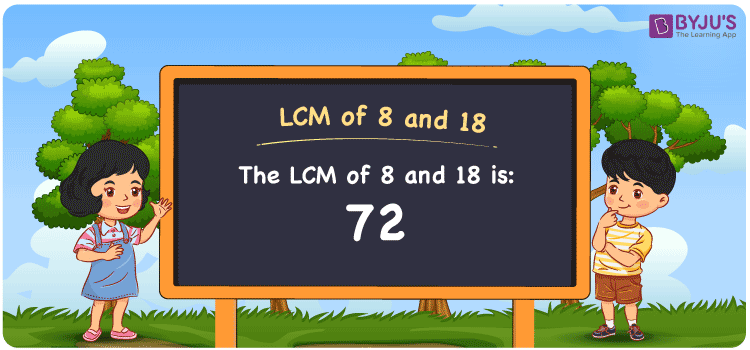Checkout JEE MAINS 2022 Question Paper Analysis : Checkout JEE MAINS 2022 Question Paper Analysis :

# LCM of 8 and 18

LCM of 8 and 18 is 72. In Mathematics, the LCM of two numbers is the value that is evenly divisible by the two given numbers. Least common multiple of 8 and 18 is the smallest number we obtain from the common multiples. (8, 16, 24, 32, 40, ….) and (18, 36, 54, 72, 90,….) are the multiples of 8 and 18. We can get the LCM easily using various methods like prime factorisation, division and listing the multiples.

## What is LCM of 8 and 18?

The answer to this question is 72. The LCM of 8 and 18 using various methods is shown in this article for your reference. The LCM of two non-zero integers, 8 and 18, is the smallest positive integer 72 which is divisible by both 8 and 18 with no remainder.## How to Find LCM of 8 and 18?

LCM of 8 and 18 can be found using three methods:

• Prime Factorisation
• Division method
• Listing the multiples

### LCM of 8 and 18 Using Prime Factorisation Method

The prime factorisation of 8 and 18, respectively, is given by:

8 = 2 x 2 x 2 = 2³

18 = 2 x 3 x 3 = 2¹ x 3²

LCM (8, 18) = 72

### LCM of 8 and 18 Using Division Method

We’ll divide the numbers (8, 18) by their prime factors to get the LCM of 8 and 18 using the division method (preferably common). The LCM of 8 and 18 is calculated by multiplying these divisors.

 2 8 18 2 4 9 2 2 9 3 1 9 3 1 3 x 1 1

No further division can be done.

Hence, LCM (8, 18) = 72

### LCM of 8 and 18 Using Listing the Multiples

To calculate the LCM of 8 and 18 by listing out the common multiples, list the multiples as shown below

 Multiples of 8 Multiples of 18 8 18 16 36 24 54 32 72 40 90 48 108 56 126 64 144 72 162 80 180

The smallest common multiple of 8 and 18 is 72.

Therefore LCM (8, 18) = 72

## Video Lesson on Applications of LCM## LCM of 8 and 18 Solved Examples

Question: What is the LCM if the product of two numbers is 144 and the GCD is 2?

Solution:

It is given that

Product of two numbers = 144

GCD = 2

We know that

LCM x GCD = Product of two numbers

LCM = Product/GCD

LCM = 144/2

LCM = 72

Hence, the LCM is 72.

## Frequently Asked Questions on LCM of 8 and 18

### What is the LCM of 8 and 18?

The LCM of 8 and 18 is 72. To determine the LCM, we should know the multiples of 8 and 18 and the smallest multiple exactly divisible by 8 and 18 has to be found.

### Mention the methods used to determine the LCM of 8 and 18.

The methods used to determine the LCM of 8 and 18 are Prime Factorization Method, Division Method and Listing multiples.

### Calculate the GCF if the LCM of 8 and 18 is 72.

LCM x GCF = 8 x 18

Given

LCM of 8 and 18 = 72

72 x GCF = 144

GCF = 144/72 = 2